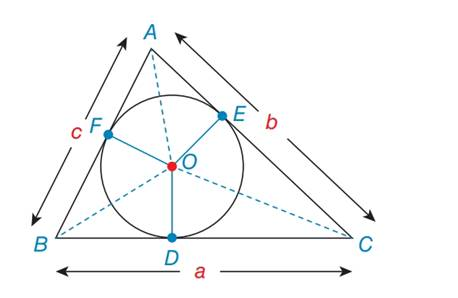Chapter 7.2, Problem 42E### Elementary Geometry for College St...

6th Edition
Daniel C. Alexander + 1 other
ISBN: 9781285195698

#### Solutions

Chapter
Section### Elementary Geometry for College St...

6th Edition
Daniel C. Alexander + 1 other
ISBN: 9781285195698
Textbook Problem
1 views

# For exercises 42 to 44, ⊙ O is the inscribed circle for ∆ A B C . In exercises 43 and 44, use the result from 42.Explain why ∆ A O F ≅ A O E .To determine

To explain:

Why ΔAOEΔAOF

Explanation

Definition:

Angle Angle Side (AAS):

If two angles and a non included side of a triangle are congruent to two angles and the corresponding non included side of another triangle, then the two triangles are congruent by angle angle side postulate.

Given:

Explain ΔAOEΔAOF

Calculation:

Consider the triangle ΔAOEandΔAOF

### Still sussing out bartleby?

Check out a sample textbook solution.

See a sample solution

#### The Solution to Your Study Problems

Bartleby provides explanations to thousands of textbook problems written by our experts, many with advanced degrees!

Get Started

#### Find more solutions based on key concepts